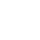#Documentation

Available on the Open Source plans.

In order to faciliate to use our products the following information is useful:

The following IaaS provider is supported.

1. OpenNebula

Configure region, cluster, network and VCPU here.

Go to

``````
\$ cd /var/lib/detio

``````

Configure /var/lib/detio/vertice/vertice.conf

``````
###
### [deployd]
###
### Controls how the deployer endpoints are configured. These are the primary mechanism to
### deploy on the cloud. The default option is to support opennebula.
###

[deployd]
provider = "one"

[deployd.one]
enabled = true
vcpu_percentage = "3"
[[deployd.one.region]]
# region name, this has to be the same as the Region configuration.
one_zone = "chennai"
# opennebula endpoint
one_endpoint = "http://localhost:2633/RPC2"
one_template = "megam"
# Virtual machine uses 10% of 1 core (ie 1VCPU = (1Core/10))
vcpu_percentage = "10"

[[deployd.one.region.cluster]]
enabled = true
#opennebula cluster
cluster_id = "101"
# storage type of node such hdd/ssd
storage_hddtype = "hdd"
# opennebula virtual network configured
vnet_pri_ipv4   = "pri_ipv4"
vnet_pub_ipv4   = "pub2_ipv4"
vnet_pri_ipv6   = "pri_ipv6"
vnet_pub_ipv6   = "pub_ipv6"

[[deployd.one.region.cluster]]
enabled = true
cluster_id = "100"
storage_hddtype = "ssd"
vnet_pri_ipv4   = "pri_ipv4-a"
vnet_pub_ipv4   = "pub_ipv4-a"
vnet_pri_ipv6   = "pri_ipv6-a"
vnet_pub_ipv6   = "pub_ipv6-a"

#one or more regions can be added
[[deployd.one.region]]
one_zone = "sydney"
one_endpoint = "http://127.0.0.1:2633/RPC2"
one_template = "megam"
vcpu_percentage = "10"

[[deployd.one.region.cluster]]
enabled = true
cluster_id = "103"
storage_hddtype = "ssd"
vnet_pri_ipv4   = "pri_ipv4-a"
vnet_pub_ipv4   = "pub_ipv4-a"
vnet_pri_ipv6   = "pri_ipv6-a"
vnet_pub_ipv6   = "pub_ipv6-a"

[[deployd.one.region.cluster]]
enabled = true
cluster_id = "104"
storage_hddtype = "hdd"
vnet_pri_ipv4   = "pri_ipv4-b"
vnet_pub_ipv4   = "pub_ipv4-b"
vnet_pri_ipv6   = "pri_ipv6-b"
vnet_pub_ipv6   = "pub_ipv6-b"
``````